# LIC AAO/SBI PO Prelims Quantitative Aptitude Questions 2019 (Day-05)

Dear Aspirants, Our IBPS Guide team is providing new series of Quantitative Aptitude Questions for LIC AAO/SBI PO 2019 so the aspirants can practice it on a daily basis. These questions are framed by our skilled experts after understanding your needs thoroughly. Aspirants can practice these new series questions daily to familiarize with the exact exam pattern and make your preparation effective.

[WpProQuiz 5518]

### Click Here for SBI PO Pre 2019 High-Quality Mocks Exactly on SBI Standard

Directions (1 – 2): A number is bolded in the series I, II and III. Find the wrong series which does not satisfies the given number and choose the options accordingly.

1)

I)18, 29, 43, 63, 101, 211

II)79, 40, 41, 63, 128, 322.5

III)53, 55, 63, 92, 157, 283

a) Both I and II

b) Both II and III

c) Only I

d) Only III

e) Only II

2)

I)15, 16, 34, 105, 424, 2125

II)108, 21, 84, 28, 56

III)17, 105, 149, 171, 182, 187.5

a) Only I

b) Both I and II

c) Only II

d) Both II and III

e) Only III

Directions (Q. 3 – 5): Find out the wrong number in the following number series?

3) 384, 192, 286, 720, 2520, 11340

a) 2520

b) 286

c) 11340

d) 384

e) None of these

4) 32, 107, 184, 262, 349, 441, 544

a) 107

b) 441

c) 32

d) 262

e) 349

5) 12, 16, 44, 180, 784

a) 44

b) 16

c) 784

d) 12

e) 180

Directions (Q. 6 – 10): Study the following information carefully and answer the given questions:

The following table shows the amount (In lakhs) earned by 5 persons in 6 different years.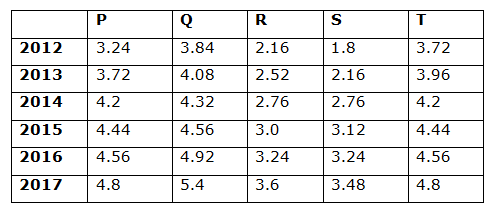6) The total amount earned by all the given persons in the year 2012 and 2014 together is approximately what percentage more/less than the total amount earned by all the given persons in the year 2016 and 2017 together?

a) 42 % more

b) 23 % more

c) 23 % less

d) 8 % more

e) 42 % less

7) Find the difference between the total amount earned by Q in all the given years together to that of total amount earned by S in all the given years together?

a) 10.56 lakhs

b) 12.34 lakhs

c) 14.78 lakhs

d) 16.22 lakhs

e) None of these

8) Find the ratio between the total amountsearned by P, Q and R in the years 2012, 2014 and 2016 together to that of total amount earned by R, S and T in the years 2013, 2015 and 2017 together?

a) 132: 119

b) 215: 176

c) 345: 247

d) 277: 259

e) None of these

9) Find the average amount earned by P, R and T together in all the given years?

a) 45.36 lakhs

b) 36.28 lakhs

c) 22.64 lakhs

d) 58.12 lakhs

e) None of these

10) The total amount earned by Q in the year 2012, 2014 and 2016 together is approximately what percentage of total amount earned by S in the year 2013, 2015 and 2017 together?

a) 150 %

b) 130 %

c) 115 %

d) 85 %

e) 165 %

Direction (1-2) :

Series I pattern: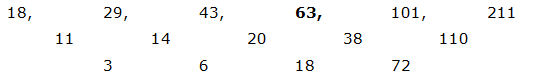The difference of difference is, *2, *3, *4,….

The bolded number is followed the given series.

Series II pattern:

79*0.5 + 0.5 = 40

40*1 + 1 = 41

41*1.5 + 1.5 = 63

63*2 + 2 = 128

128*2.5 + 2.5 = 322.5

The bolded number is followed the given series.

Series III pattern: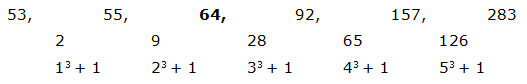The difference is, 13 + 1, 23 + 1, 33 + 1, 43 + 1, 53 + 1,….

The bolded number is not followed the given series.

Series I pattern:

15*1 + 1 = 16

16*2 + 2 = 34

34*3 + 3 = 105

105*4 + 4 = 424

424*5 + 5 = 2125

The bolded number is followed the given series.

Series II pattern:

105 ÷ 5 = 21

21*4 = 84

84 ÷ 3 = 28

28*2 = 56

The bolded number is not followed the given series.

Series III pattern: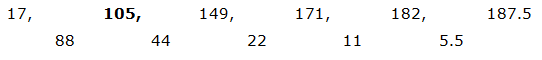The pattern is, the difference of two numbers ÷ 2

The bolded number is followed the given series.

The correct series is,

384, 192, 288, 720, 2520, 11340

The pattern is, *1/2, *3/2, *5/2, *7/2, *9/2,….

The wrong term is, 286

The correct series is,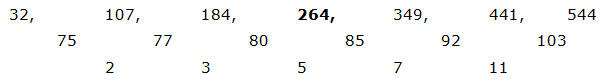The difference of difference is, 2, 3, 5, 7, 11,…. (Prime numbers)

The wrong term is, 262

The correct series is,

12, 16, 48, 180, 784

The pattern is, *1 + 22, *2 + 42, *3 + 62, *4 +82,…..

The wrong term is, 44

Direction (6-10) :

The total amount earned by all the given persons in the year 2012 and 2014 together

= > 3.24 + 3.84 + 2.16 + 1.8 + 3.72 + 4.2 + 4.32 + 2.76 + 2.76 + 4.2

= > 33 lakhs

The total amount earned by all the given persons in the year 2016 and 2017 together

= > 4.56 + 4.92 + 3.24 + 3.24 + 4.56 + 4.8 + 5.4 + 3.6 + 3.48 + 4.8

= > 42.6 lakhs

Required % = [(42.6 – 33)/42.6]*100 = 22.53 % = 23 % less

The total amount earned by Q in all the given years together

= > 3.84 + 4.08 + 4.32 + 4.56 + 4.92 + 5.4

= > 27.12 lakhs

The total amount earned by S in all the given years together

= > 1.8 + 2.16 + 2.76 + 3.12 + 3.24 + 3.48

= > 16.56 lakhs

Required difference = 27.12 – 16.56 = 10.56 lakhs

The total amount earned by P, Q and R in the year 2012, 2014 and 2016 together

= > 3.24 + 3.84 + 2.16 + 4.2 + 4.32 + 2.76 + 4.56 + 4.92 + 3.24

= > 33.24 lakhs

The total amount earned by R, S and T in the year 2013, 2015 and 2017 together

= > 2.52 + 2.16 + 3.96 + 3.0 + 3.12 + 4.44 + 3.6 + 3.48 + 4.8

= > 31.08 lakhs

Required ratio = 33.24: 31.08 = 277: 259

The total amount earned by P, R and T together in all the given years

= > 3.24 + 3.72 + 4.2 + 4.44 + 4.56 + 4.8 + 2.16 + 2.52 + 2.76 + 3.0 + 3.24 + 3.6 + 3.72 + 3.96 + 4.2 + 4.44 + 4.56 + 4.8

= > 67.92 lakhs

Required average = 67.92/3 = 22.64 lakhs

The total amount earned by Q in the year 2012, 2014 and 2016 together

= > 3.84 + 4.32 + 4.92 =13.08 lakhs

The total amount earned by S in the year 2013, 2015 and 2017 together

= > 2.16 + 3.12 + 3.48 = 8.76 lakhs

Required % = (13/08/8.76)*100 = 149.31 % = 150 %